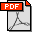J. Gao, L. Guibas, S. Oudot, and Y. Wang. Geodesic Delaunay Triangulation and Witness Complex in the Plane. Proc. 19th ACM-SIAM Symposium on Discrete Algorithms, pages 571-580, 2008. Full version invited to the special issue of Transactions on Algorithms on SODA'08 (full version). Abstract: We introduce a novel feature size for bounded planar domains endowed with an intrinsic metric. Given a point x in such a domain X, the systolic feature size of X at x, or sfs(x) for short, measures half the length of the shortest loop through x that is not null-homotopic in X. The resort to an intrinsic metric makes sfs(x) rather insensitive to the local geometry of X, in contrast with its predecessors (local feature size, weak feature size, homology feature size). This leads to a reduced number of samples that still capture the topology of X. Under reasonable sampling conditions involving sfs, we show that the geodesic Delaunay triangulation D_X(L) of a finite sampling L of X is homotopy equivalent to X. Moreover, D_X(L) is sandwiched between the geodesic witness complex W_X (L) and a relaxed version W^lambda_X(L), defined by some relaxation parameter lambda. Taking advantage of this fact, we prove that the homology of D_X(L) (and hence of X) can be retrieved by computing the persistent homology between W_X (L) and W^lambda_X(L). Our proofs draw some connections with recent advances on the front of homology inference from point cloud data, but also with several well-known concepts of Riemannian (and even metric) geometry. On the algorithmic front, we propose algorithms for estimating sfs, selecting a landmark set of sufficient density, building its geodesic Delaunay triangulation, and computing the homology of X using W_X(L) and W^lambda_X(L). We also present some simulation results in the context of sensor networks that corroborate our theoretical claims. Bibtex: ```@inproceedings{ggow-gtwcp-08 , author = {J. Gao and L. Guibas and S. Oudot and Y. Wang} , title = {Geodesic Delaunay Triangulation and Witness Complex in the Plane} , booktitle = {Proc. 18th ACM-SIAM Sympos. on Discrete Algorithms} , pages = {571--580} , year = {2008} } ```